# Definitions and some radiation theory¶

In radiation physics there is unfortunately several slightly different ways of presenting the theory. For instance, there is no single custom on the mathematical symbolism, and various different non SI-units are used in different situations. Here we present just a few terms and definitions with relevance to PySpectral, and how to possible go from one common representation to another.

## Symbols and definitions used in PySpectral¶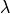Wavelength (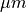)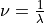Wavenumber (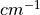)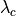Central wavelength for a given band/channel ()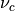Central wavelength for a given band/channel ()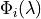Relative spectral response for bandas a function of wavelength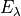Spectral irradiance at wavelength(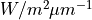)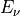Spectral irradiance at wavenumber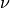(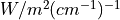)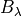Blackbody radiation at wavelength()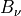Blackbody radiation at wavenumber()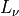Spectral radiance at wavenumber(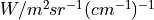)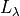Spectral radiance at wavelength(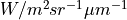)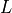Radiance - (band) integrated spectral radiance (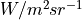)

## Constants¶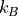Boltzmann constant (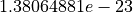)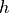Planck constant (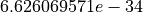)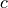Speed of light in vacuum (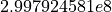)

## Central wavelength and central wavenumber¶

The central wavelength for a given spectral band () of a satellite sensor is defined as: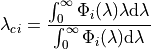Likewise the central wavenumber is: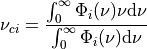And since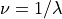, we can express the central wavenumber using the spectral response function expressed in wavelength space, as: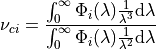And from this we see that in general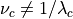.

Taking SEVIRI as an example, and looking at the visible channel on Meteosat-8, we see that this is indeed true:

>>> from pyspectral.rsr_reader import RelativeSpectralResponse
>>> from pyspectral.utils import convert2wavenumber, get_central_wave
>>> seviri = RelativeSpectralResponse('Meteosat-8', 'seviri')
>>> cwl = get_central_wave(seviri.rsr['VIS0.6']['det-1']['wavelength'], seviri.rsr['VIS0.6']['det-1']['response'])
>>> print(round(cwl, 6))
0.640216
>>> rsr, info = convert2wavenumber(seviri.rsr)
>>> print("si_scale={scale}, unit={unit}".format(scale=info['si_scale'], unit=info['unit']))
si_scale=100.0, unit=cm-1
>>> wvc = get_central_wave(rsr['VIS0.6']['det-1']['wavenumber'], rsr['VIS0.6']['det-1']['response'])
>>> round(wvc, 3)
15682.622
>>> print(round(1./wvc*1e4, 6))
0.637648


In the PySpectral unified HDF5 formatted spectral response data we also store the central wavelength, so you actually don’t have to calculate them yourself:

>>> from pyspectral.rsr_reader import RelativeSpectralResponse
>>> print("Central wavelength = {cwl}".format(cwl=round(seviri.rsr['VIS0.6']['det-1']['central_wavelength'], 6)))
Central wavelength = 0.640216


## Wavelength range¶

Satellite radiometers do not measure outgoing radiance at only the central wavelength, but over a range of wavelengths defined by the spectral response function of the instrument. A given instrument channel is therefore often defined by a wavelength range in the form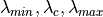. Where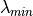and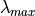are defined by the first and last points in the spectral response function where the response is greater than a given threshold. Pyspectral has a utility function to enable users to compute these wavelength ranges:

>>> from pyspectral.rsr_reader import RelativeSpectralResponse
>>> from pyspectral.utils import get_wave_range
>>> seviri = RelativeSpectralResponse('Meteosat-8', 'seviri')
>>> wvl_range = get_wave_range(seviri.rsr['HRV']['det-1'], 0.15)
>>> print(round(num, 3) for num in wvl_range)
[0.456, 0.715, 0.996]


We denote the spectral irradiance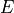which is a function of wavelength or wavenumber, depending on what representation is used. In PySpectral the aim is to support both representations. The units are of course dependent of which representation is used.

In wavelength space we write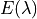and it is given in units of.

In wavenumber space we write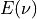and it is given in units of.

To convert a spectral irradiance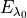at wavelength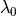to a spectral irradiance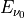at wavenumber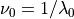the following relation applies: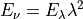And if the units are not SI but rather given by the units shown above we have to account for a factor of 10 as: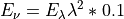## TOA Solar irradiance and solar constant¶

First, the TOA solar irradiance in wavelength space:

>>> from pyspectral.solar import (SolarIrradianceSpectrum, TOTAL_IRRADIANCE_SPECTRUM_2000ASTM)
>>> print("Solar irradiance = {}".format(round(solar_irr.solar_constant(), 3)))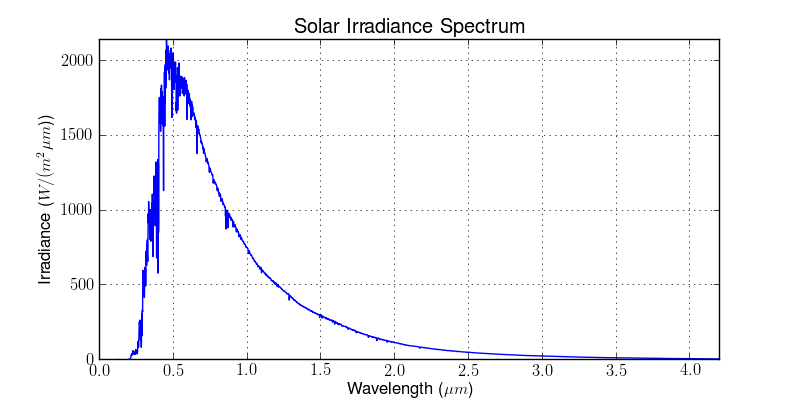The solar constant is in units of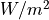. Instead when expressing the irradiance in wavenumber space using wavenumbers in units ofthe solar flux is in units of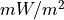:

>>> solar_irr = SolarIrradianceSpectrum(TOTAL_IRRADIANCE_SPECTRUM_2000ASTM, dlambda=0.0005, wavespace='wavenumber')
>>> print(round(solar_irr.solar_constant(), 5))
1366077.16482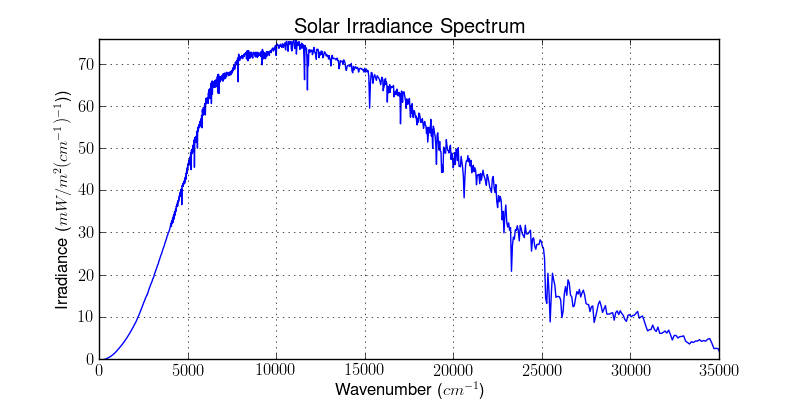## In-band solar flux¶

The solar flux (SI unit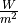) over a spectral sensor band can be derived by convolving the top of atmosphere solar spectral irradiance and the sensor relative spectral response. For band: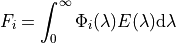whereis the TOA spectral solar irradiance at a sun-earth distance of one astronomical unit (AU).

In python code it may look like this:

>>> from pyspectral.rsr_reader import RelativeSpectralResponse
>>> from pyspectral.utils import convert2wavenumber, get_central_wave
>>> seviri = RelativeSpectralResponse('Meteosat-8', 'seviri')
>>> rsr, info = convert2wavenumber(seviri.rsr)
>>> print("Solar Irradiance (SEVIRI band VIS008) = {sflux:12.6f}".format(sflux=solar_irr.inband_solarflux(rsr['VIS0.8'])))
Solar Irradiance (SEVIRI band VIS008) = 63767.908405


Planck’s law describes the electromagnetic radiation emitted by a black body in thermal equilibrium at a definite temperature.

Thus for wavelengththe Planck radiation or Blackbody radiation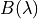can be written as: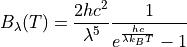and expressed as a function of wavenumber: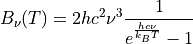In python it may look like this:

>>> from pyspectral.blackbody import blackbody_wn
>>> wavenumber = 90909.1
>>> rad = blackbody_wn((wavenumber, ), [300., 301])
0.001158 0.001175


Which are the spectral radiances in SI units at wavenumber around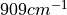at temperatures 300 and 301 Kelvin. In units of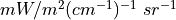this becomes:

>>> print("{0:7.4f} {1:7.4f}".format((rad*1e+5), (rad*1e+5)))
115.8354 117.5477


And using wavelength representation:

>>> from pyspectral.blackbody import blackbody
>>> wvl = 1./wavenumber
>>> rad = blackbody(wvl, [300., 301])
9573177.494 9714687.157


Which are the spectral radiances in SI units around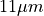at temperatures 300 and 301 Kelvin. In units of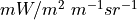this becomes:

>>> print("{0:7.5f} {1:7.5f}".format((rad*1e-6), (rad*1e-6)))
9.57318 9.71469


## The inverse Planck function¶

Inverting the Planck function allows to derive the brightness temperature given the spectral radiance. Expressed in wavenumber space this becomes: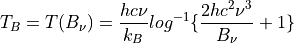With the spectral radiance given as a function of wavelength the equation looks like this: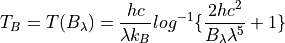In python it may look like this:

>>> from pyspectral.blackbody import blackbody_wn_rad2temp
>>> wavenumber = 90909.1
>>> temp = blackbody_wn_rad2temp(wavenumber, [0.001158354, 0.001175477])
>>> print([round(t, 8) for t in temp])
[299.99998562, 301.00000518]


This approach only works for monochromatic or very narrow bands for which the spectral response function is assumed to be constant. In reality, typical imager channels are not that narrow and the spectral response function is not constant over the band. Here it is not possible to de-convolve the planck function and the spectral response function without knowing both, the spectral radiance and spectral response function in high spectral resolution. While this information is usually available for the spectral response function, there is only one integrated radiance per channel. That makes the derivation of brightness temperature from radiance more complicated and more time consuming - in preparation or in execution. Depending on individual requirements, there is a bunch of feasible solutions:

### Iterative Method¶

A stepwise approach, which starts with a guess (most common temperature), calculate the radiance that would correspond to that temperature and compare it with the measured radiance. If the difference lies above a certain threshold, adjust the temperature accordingly and start over again:

1. set uncertainty parameter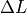2. set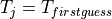3. calculate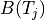4. if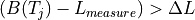then adjust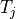and go back to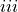5.matches the measurement within the defined uncertainty

• no pre-computations

• accuracy easily adaptable to purpose

• memory friendly

• independent of band

• independent of spectral response function

• slow, especially when applying to wide bands and high accuracy requirements

• redundant calculations when applying to images with many pixels

### Function Fit¶

Another feasible approach is to fit a function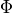in a way that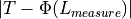minimizes. This requires pre-calculations of data pairs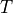and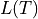. Finally an adequate function(dependent on the shape of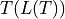) is assigned and used to calculate the brightness temperature for one channel.

• fast approach (especially in execution)

• minor memory request (one function per channel)

• accuracy determined in the beginning of the process

• complexity ofdepends on### Look-Up Table¶

If the number of possible pairsandis limited (e.g. due to limited bit size) or if the setting for a function fit is too complex or does not fit into a processing environment, it is possible to just expand the number of pre-calculated pairs to a look-up table. In an optimal case, the table cover every possible value or is dense enough to allow for linear interpolation.